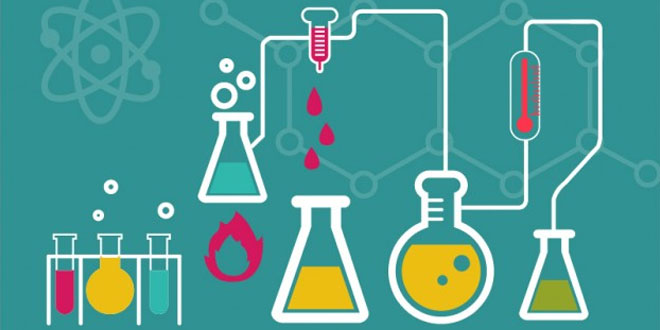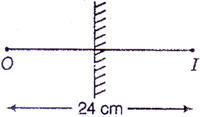Sunday , January 17 2021# 8th Science Periodic Test II (2018-19)

## 8th Science Periodic Test II (2018-19)

School Name: Venkateshwar Global School, Sector 13, Rohini, Delhi 110085 India
Time: 3 hour
M.M. 80 marks
Date: 24/09/2018
Class: VIII

## General Instructions:

1. All Questions are compulsory.
3. Draw the diagrams neatly only with a pencil.

### Short answer questions (I) (2×6 = 12 marks)

#### Question: 8. The distance between the object and its image formed by a plane mirror appears to be 24 cm. What is the distance between the mirror and the object?### Short answer question (II): (3×10 = 30 marks)

#### Question: 10. Give reasons:

1. Aluminium foil is used to wrap food items
2. Silver articles corrode easily now a days.
3. There is a difference in sound upon dropping a metal coin and piece of coal.

#### Question: 11. Explain why:

2. Deep sea divers wear special suits.

#### Question: 19. Ritu rows at boat with a force of 700 N while running water applies a force of 500 N.  Calculate the following.

1. Resultant force while going downstream (the direction of the river add boat is the same)
2. Resultant force while going upstream (the direction of the river is opposite to the direction in which the boat is moving).

### Long answer questions: (5×7 = 35 marks)

#### Question: 21.

1. Why is nitrogen gas filled in chips packets?
2. Who is closer to plants  an algae or a protozoan? Why?
3. We should complete the course of antibiotics prescribed by the physician. What would happen if we leave the course midway?

#### Question: 23. Explain with the help of a chemical reaction what happens when:

1. Aluminium is added to copper sulphate solution.
2. Iron reacts with oxygen and moist air.
3. Copper reacts with magnesium sulphtae.
4. Potassium reacts with water.
5. Iron reacts with steam.

#### Question: 24.

1. Discuss three situations where friction is disadvantageous.
2. Give two methods by which friction between two surfaces in contact can be reduced.

## 11th CBSE NCERT Computer Science Mid Term Exam Set A

11th Class CBSE NCERT Computer Science: Mid Term Examination Set – A (2019-20) Time: 3 Hrs. …